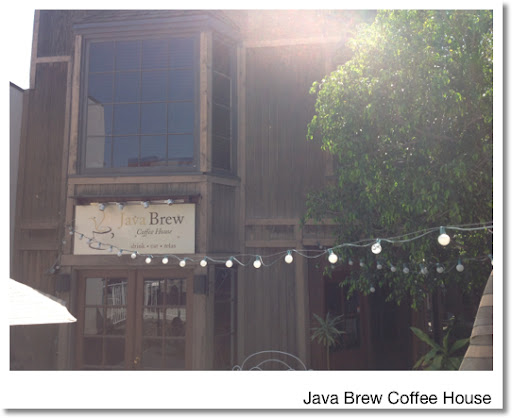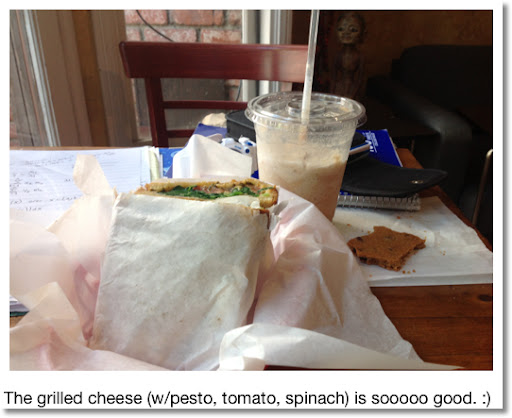## Saturday, April 27, 2013

### Java Brew Coffee House and Other Saturday Adventures

I hope everyone is doing well today! I am coming to you form Java House Coffee Shop in Montrose, CA.

This morning I went to the Glendale College Library. Guests have to park on the top lot. To get to the campus required a combination stair climbing and taking elevators. I love the view from the top.

Among subjects studied today are centriods, inverse problems, and surface integrals. Three hours went by and I left 20 minutes before the library closed, which only proves the saying "So much to do, and so little time."

Here is some of what studied today:
------
Centriods:

Points (x,y) each with mass m:

X-bar: Σ(x*m)/Σm, Y-bar: Σ(y*m)/Σm

Continuous functions, uniform thickness and density, f(x) ≥ g(x), limits a ≤ x ≤ b,

M_y = ∫(x * (f(x) - g(x)) dx, a, b)
M_x = 1/2 * ∫( (f(x))^2 - (g(x))^2, x, a, b)
M = ∫( f(x) - g(x) dx, a, b)

X-bar: M_y/M, Y-bar: M_x/M

Jon Guilleberg. "Mathematics: From the Birth of Numbers." W.M. Norton & Co. 1997

Normal Vectors

If a vector is perpendicular to another vector, the former vector is known as a normal vector. We can find a normal vector to any two vectors U and V by the cross product.

N = U × V

And the Unit Normal Vector:

n = (U × V)/|U × V| = vector/(norm of a vector)

Applying the normal unit vector to a said function z = f(x,y):

n = [-df/dx, -df/dy, 1] / √( 1 + (df/dx)^2 + (df/dy)^2 )

A unit vector has length 1.

Pretend that "d" is the partial derivative operator.

Surface area of z = f(x,y) over region R is:

∫ ∫ (√( 1 + (df/dx)^2 + (df/dy)^2 ) dx dy

H.M. Schey "div grad curl and all that" W.M. Norton & Co. 1992

Yes the title was in all lowercase. I get weirdly fascinated by all-lowercase titles.Going to finish my lunch, and do some programming. Love to all of you!

Eddie

This blog is property of Edward Shore. 2013